# Integral of cos(x)

In this post we saw the most important integrals to memorize in order to solve more complex ones. One of them is the integral of cos(x), which is sin(x). But why?

Definition of cos(x)

We can define cos(x) using exponential functions and, in particular, Euler’s identity: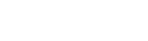e is Euler’s number and i is the imaginary unit.; e^(ix) is Euler’s identity: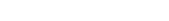We can prove cos(x) can be expressed as shown above: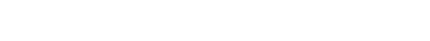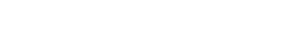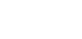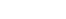Integral of cos(x)

Now that we know that the two expressions are equivalent, we can confirm that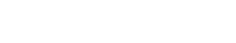and we know how to evaluate the second integral: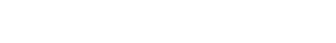Linearity and constant rule are applied and now we have two integrals, very easy to solve using u-substitution. You actually don’t need to perform u-sub: just rewrite the function and divide it by the exponent without the x: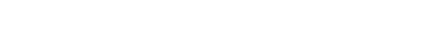Don’t forget to add the +C at the end. I didn’t write it to isolate the result. If you really want to use u-sub, simply let u=ix for the first integral and u=-ix for the second; you will see that it’s the exact same thing.

Anyway, that’s the result. Let me tell you that sin(x) can be written as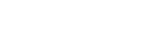This is the expression we got from the integral! So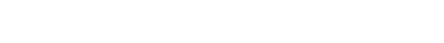This is a demostration why the integral of cos(x) is sin(x).

Join 31 other subscribers.